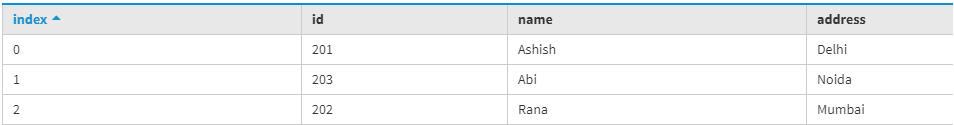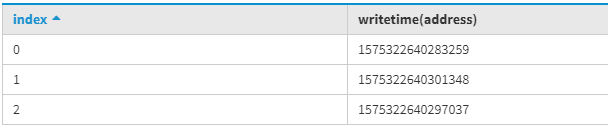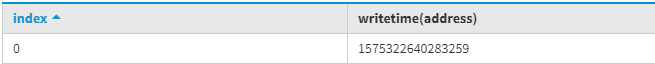Related Articles
Additional Functions in CQL (Cassandra Query Language)
• Last Updated : 05 Dec, 2019

In this article, we will discuss several functions that are supported by CQL in Cassandra which can help in converting one value type into another new value directly by using the function.
In Cassandra, there are several functions that are supported by CQL which helps in many ways such that there is a scenario where we want to find the TTL value of a column with the help of TTL function we can easily do that.

1. Aggregate function
2. UUID and timeuuid function
3. TOKEN function
4. WRITETIME function
5. TTL function

Let’s discuss WRITETIME function.

WRITETIME :
The WRITETIME function is very useful in Cassandra query language when write occurred then we can retrieve the date/time of writes to the columns.
We can use WRITETIME function in the select statement followed by the non-partitioning column in parenthesis. In Cassandra Query Language a table contains the timestamp to represents date and time that a write occurred to a column. After return the query it gives WRITETIME function value in microseconds and then we can convert it into date/time formats.

Let’s understand with an example.

```CREATE TABLE function_test
(
Id int,
Name text,
PRIMARY KEY(Id)
);```

To insert data into the table used the following CQL query.

```INSERT INTO function_test (Id, Name, Address)
VALUES (201, 'Ashish', 'Delhi');
INSERT INTO function_test (Id, Name, Address)
VALUES (202, 'Rana', 'Mumbai');
INSERT INTO function_test (Id, Name, Address)
VALUES (203, 'Abi', 'Noida'); ```

To read the data used the following CQL query.

```SELECT *
FROM function_test; ```

Output:To determine the WRITETIME function Value used the following CQL query.

```SELECT WRITETIME (Address)
FROM function_test; ```

Output:To determine the WRITETIME function by using WHERE clause used the following CQL query.

```SELECT WRITETIME (Address)
FROM function_test where Id=201; ```

Output:My Personal Notes arrow_drop_up